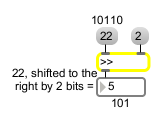>>

Shift all bits to the right

Description

>> has the effect of dividing a number by a power of two, but is less expensive in computer time.

Arguments

Name Type Opt Description
initial-value int opt Sets an initial value for the number of bits by which to shift rightward.

Messages

 bang In left inlet: Performs the bit-shift with the numbers currently stored. If there is no argument, >> initially holds 0 as the number of bits by which to shift. int input [int] In left inlet: All bits of the number, in binary form, are shifted to the right by a certain number of bits. The resulting number is sent out the outlet. (inlet1) amount-of-bitshift [int] In right inlet: The number is stored as the number of bits to right-shift the number in the left inlet. float input [float] Converted to int. set set-input [int] Sets input to the object without causing output (bang will output it). list input [list] In left inlet: The first number is bit-shifted to the right by the number of bits specified by the second number.

Output

int: The number in the left inlet is bit-shifted to the right by a certain number of bits. The number of bits by which to shift is specified by the number in the right inlet. The output is the resulting bit-shifted number.

ExamplesSame effect as dividing by a power of 2